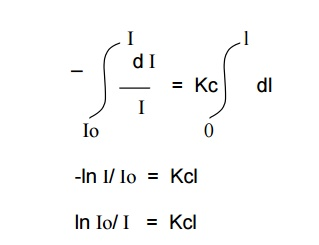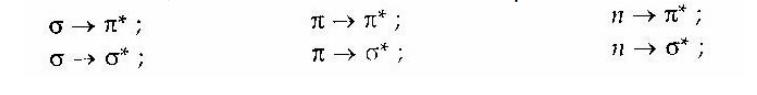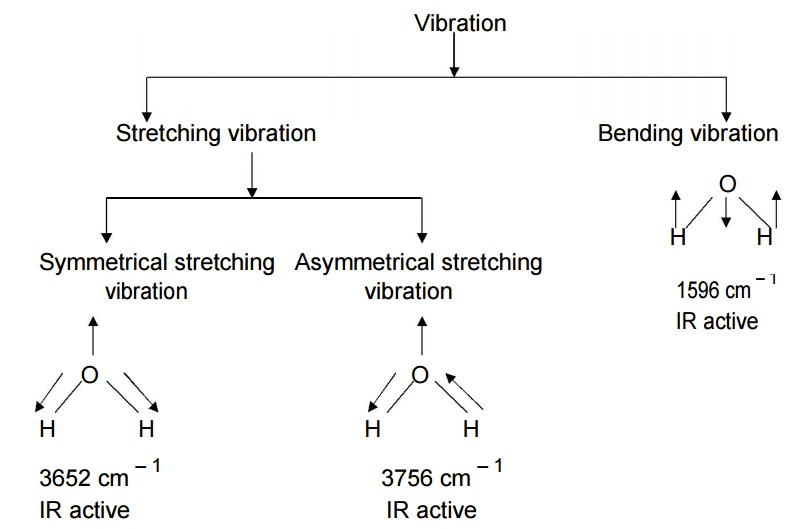Home | | Engineering Chemistry I | Spectroscopy

# Spectroscopy

Analytical chemistry is concerned with the identification of a substance, the elucidation of its structure and quantitative analysis of its composition.

Spectroscopy

ANALYTICAL CHEMISTRY

Analytical chemistry is concerned with the identification of a substance, the elucidation of its structure and quantitative analysis of its composition.

It is an interdisciplinary branch of science which deals with various disciplines of chemistry such as inorganic, organic, physical, industrial and biochemistry

INSTRUMENTAL METHODS

The methods dependent upon measurement of an electrical property and those based upon determination of the extent to which radiation is absorbed or upon assessment of the intensity of emitted radiation, all require the use of a suitable instrument, eg. Polarograph, spectrophotometer etc., and in consequence such methods are referred to as instrumental methods.

The growth of instrumental methods (or) analysis is related to the developments in the field of electronics, so instrumental methods of chemical analysis have become the backbone of experimental chemistry.

ANALYTICAL TECHNIQUES –SPECTROSCOPY

Spectroscopy is the branch of science dealing with the study of interaction of electromagnetic radiation with matter.

It is a powerful tool available for the study of atomic and molecular structure and it is also used in the analysis of most of the samples.

Types of Spectroscopy:-

The study of spectroscopy is divided into two types. They are,

1.     Atomic spectroscopy

2.     Molecular spectroscopy.

Atomic Spectroscopy

It deals with the interactions of electromagnetic radiation with atoms.

Molecular Spectroscopy

It deals with the interaction of electromagnetic radiation with molecules.

Differences between atomic spectra and molecular spectraAtomic Spectra

1. It occurs from the interaction of atoms and electromagnetic radiation.

2. It is a line spectra

3.It is a due to electronic transition in an element

Molecular Spectra

1. It occurs from the interaction of molecules and electromagnetic radiation

2. It is a complicated spectra.

3. It is due to vibrational, rotational and electronic transition in a molecule

Electromagnetic radiation is produced due to the interaction between the electric field and the magnetic field.

Electromagnetic radiation is divided into number of regions according to their wave length.

It can be represented as,

λ        (lambda)Wave length (λ)

It is the distance between two successive crests of a wave.

It is denoted by ‘λ’. Wave length is express lengths nanometer (nm,10-9m), picometer (pm,10-12m) or ‘non SI’ Units Angstrom (Ao,10-10m)Frequency (ע)

It is the number of waves crossing a point in unit time. It is denoted by, ע

Frequency is expressed in s-1(or) Hertz, (Hz).Velocity of light ( c )

The product of the wavelength and the frequency is a constant called the velocity of light (or) speed of light. That is,

C = λ

Where,

c - speed of light;

ע- frequency;

λ- wave length

c = 2.998 X 108 ms-1

c = 3 X 108 ms-1

Wave number (Bar ע -)

It is the reciprocal of the wave length. It is denoted byWave number is expressed in units of per centimeter,

Electromagnetic spectrum (EMS)

The arrangement of all types of electromagnetic radiations in the increasing order of their wavelength or decreasing order of their frequency is known as ‘Electromagnetic Spectrum’.PRINCIPLE OF ATOMIC SPECTROSCOPY

Spectrum

When a beam of polychromatic light is passed through a prism or grating it splits up into seven different colour. The set of colours thus obtained is called a spectrum.

The complete spectrum may extend from gamma rays of wave length 10-13 m to radiowaves of wavelength 105m.

Classification of spectra

There are two main classes of spectra namely,

1.     Absorption Spectrum.

2.     Emission Spectrum.

Absorption spectrum

When white light (ie.having all the wave length) is passed through an absorbing substance and then observed through a spectroscope, it is found that certain colours (or) wave lengths are missing and dark lines appear at their places. The specrtrum so obtained is called absorption spectrum.Emission spectrum

When the light emitted by a substance is passed through a prism and examined directly with a spectroscope, the spectra obtained is referred to as emission spectrum.Absorption Laws

There are two laws which govern the absorption of light by the molecules.

These are,

1. Lambert’s law

2. Beer’s law.

Lambert’s Law

When a beam of monochromatic light is passed through a solution of an absorbing substance, the rate of decrease of intensity of radiation (‘dI’) with thickness of the absorbing solution (‘dx’) is directly proportional to the intensity of incident radiation (I).

Mathematically, the law is expressed as,Where,

k = absorption co-efficient.

On integrating the above expression between the limits,

I = Io  at x = 0 and I = I at x = l

We get-ln I/ Io = Kl

ln Io/ I = Kl

Kl  =  ln Io/ I

By taking natural logarithm,

Kl =  2.303 log Io / I

Beer’s Law

When a beam of monochromatic light is passed through a solution of an absorbing substance, the rate of decrease of intensity of radiation (‘dI’ ) with thickness of absorbing solution (‘dx’) is directly proportional to the intensity of incident radiation (I), as well as concentration of the solution( c).

Mathematically, it is expressed asWhere,

K = Absorption co-efficient.

On integrating the above expression between the limits,

I = Io at x = 0

I = I at x = l

We get,By taking natural logarithms,

2.303 log Io/ I  = Kcl

log Io/ I   = K / 2.303 . cl

log Io/ I   =  εcl

where,

ε K=/2.303, is called the molar absorption coefficient and

log Io/ I  = A, is called the absorbance

A = εcl

This equation is known as Beer – Lambert’s Law

Limitations of Beer – Lambert’s Law

This law is not obeyed if the radiation used in polychromatic.

1.     It is applicable only for dilute solutions.

2.     It is not applied to suspensions.

3.     Deviation may occur, if the solution contains impurities.

UV – Visible Spectroscopy:

Absorption radiation in the UV (wave length range = 200 – 400 nm) and Visible (wave length range = 400 – 750 nm) regions of the electromagnetic spectrum results transitions between electronic levels. This is due to the larger energy change corresponds to 100 – 100,000 kJ/mol which cause simultaneous

change in vibrational and rotation energies.

E elec  > E vib  > E rot

Generally energy change due to electronic transition is greater than that of vibrational and rotational transitions. Hence the UV and Visible spectra of simple molecules exihibit narrow absorption peaks.Permitted energy levels in UV and Visible regions.

The vibrational and rotational fine structure lines are not observed during the spectrum is run in solution. This is because of the physical interactions between sample organic molecule and the solvent molecule, cause collisional broadening of the lines.Electronic absorption spectrum of a solution of benzene in hexane.

The above spectra of benzene shows larger peaks at 250 nm due to the presence of П electronsaks &andtroughssmallerindicatevibrationspeofmolecules.

Types of electrons in organic molecules involving in transitions:

1.     σ electrons.

2.     П electrons.

3.     Non – bonding electrons.

According to Molecular orbital theory, the interaction of atomic orbitals leads to the formation of “bonding” and “anti – bonding” molecular orbitals. The relative energies of bonding, anti – bonding and non – bonding molecular orbitals are given in the following diagram.Bonding and antibonding orbitals.

Molecules absorb radiation from UV – Visible region and undergo various transitions. During this transition,– an bonding orbitals get excited to vacant possible excitations, there are various transitions are possible as follows.These transitions are classified into two types as follows:

1.Allowed transition:2.Forbidden transition:The order of transition isOut of the above possible transitions, the last three ones account for the absorption in 200

– 800 nm region of electromagnetic radiation and first three transitions requires much higher energy, thus the molecules characteristic spectra in the region 200 – 800 nm of electromagnetic region.

Chromophores:

The structural units of the compound having n or selective wavelength of UV – Visible radiation are called chromophores.

Example: - N=N- , C=C, C=O, etc.

Auxochromes:

The polar groups with lone pair of electrons support the intensity of chromophores are called auxochromes.

Example: ..

-O – H , - O – R , etc.

..Absorption and intensity shifts.

Bathochromic shift:

It is also called red shift. The substitution of a selective group in a molecule makes the absorption to longer wavelength is called bathochromic shift. Example: Alkyl substitution on olefins.

Hypsochromic shift:

It is also called blue shift. The substitution of a selective group in a molecule make the absorption to shorter wavelength is called hypsochromic shift. Example: Chlorine substitution on olefins.

Hyperchromic effect:

The substitution of a selective group in a molecule causes increase in the intensity of absorption maximum of the molecule, and then the effect is called hyperchromic effect. Example: Methyl substitution on benzene.

Hypochromic effect:

The substitution of a selective group in a molecule causes decrease in the intensity of absorption maximum of the molecule, and then the effect is called hypochromic effect. Example: Chlorine substitution on benzene.

UV – Visible Spectrophotometer:

Instrumentation:Block diagram for a UV – Visble spectrophotometer.

Components:

Hydrogen or deuterium lamps are used. It provides stable, continuous and sufficient intensity.

Filter:

It is also called monochromator. It permits the radiation of required wavelength

only.

Cell:

It is a transparent and uniformly constructed container which contains either sample solution or reference solvent.

Detectors:

It converts the absorbed radiation into current. There are three types of detectors, viz., Barrier layer cell, photo multiplier tube and photo cell.

Recorder: It converts the signal reaches to itself into spectrum of a molecule.

Working:

The radiation from the source is passed through the monochromator where it splitted into two equal beams, one half is passed into the sample cell and another half is passed into the reference cell containing solvent. The detector will measure the comparison of intensities of beam of light. If the sample absorbs light then the intensity of sample beam is less than the intensity of reference beam. It will be recorded as a signal in recorder. The instruments gives output graph (absorption spectrum) of a plot of the wavelength verses absorbance (A) of the light at each wavelength, where A = log (I0/I).UV – Visble spectra : (a) benzene in ethanol                (b) naphthalene in methanol.

Applications of UV spectroscopy:

1.     It is used to determine the structure of vitamins, detecting steric hindrance, study rates of reactions and determine the dissociation constants of acids and bases from the change of absorption spectra with pH.

2.     It is used to determine the dissociation energy of a molecule accurately from the wavelength.

3.     It provides the information regarding moment of inertia, vibrational frequency and interatomic distances of diatomic molecules.

4.     It is used to identify the cis and trans isomers of a compound from absorption spectra.

5.     It is used to know the purity of a compound.

6.     It is used to determine the structure of organic compounds. For example, isatin can be assigned following two possible structures:However, the corresponding structures of two possible methyl ethers are known to us.On comparison of the spectra of isatin with that of two methyl isomers (III) and (IV), the spectrum of isatin is similar to that of N-methyl ether (III). Hence isatin is assigned the structure (I).

It is used in quantitative analysis to determine the concentration of unknown sample by using Beer – Lambert’s equation, A = E c l.

IR spectroscopy:

The spectra of a molecule arised in IR region (12500 cm – 1 – 50 cm – 1 ) due to the absorption of energy and transition occurs between different vibrational levels. Hence it is called vibration spectroscopy.All types of molecules cannot interact with IR radiation. Only those molecules which exhibit change in dipole moment during a vibration can exhibit IR spectra.

Evidently, the homo-nuclear diatomic molecules like H2, O2, N2, Cl2, etc do not show change in dipole moment during vibration. Consequently, these do not exhibit IR

spectra. The hetero- nuclear diatomic / polyatomic molecules like HCl, BeCl2, NH3, CH4, CO2, C6H6, etc shows change in dipole moment and thus they exhibit IR spectra.

The IR spectral region at 1400 cm – 1 – 700 cm – 1 gives rich, intense and clear absorption bands for all functional groups in the organic compounds. This region is called finger – print region. It is used to identify the functional group present in the organic compound, Identify the molecule and find out the characteristics of the molecule.

The IR spectral region at 4000 cm – 1 – 600 cm – 1 gives intense absorption bands associated with bending and stretching vibrations of particular functional group in organic compounds. This region is called group frequency region. It is used to identify the types of functional groups present in organic molecules.

The molecules have certain number of vibrational modes. It can be calculated using the following formulae.

(a)For a linear molecule, No of fundamental vibrational mode = 3n – 5

(b)For a non – linear molecule, No of fundamental vibrational mode = 3n – 6 where n = number of atoms in a molecule.Stretching and bending vibrations in water molecule:

Water molecule has non – linear structure. It has three fundamental vibrational modes which are corresponding to the frequencies 3652 cm – 1 (Symmetric stretching vibration), 3756 cm – 1 (Asymmetric stretching vibration) and 1596 cm – 1 (Bending vibration) respectively.Generally stretching frequency is greater than bending frequency, because more energy is required to stretch the bond than to bend. All the above three vibrations are IR active and giving IR spectra at various frequencies. Hence IR active molecule undergoes change in dipole moments.

Stretching and bending vibrations in water molecule:

Carbon dioxide molecule has linear structure. It has four fundamental vibrational modes which are corresponding to the frequencies 1340 cm – 1 (Symmetric stretching vibration), 2350 cm – 1 (Asymmetric stretching vibration) and twice 666 cm – 1 (In plane bending vibration and Out of plane bending vibration) respectively.In symmetrical stretching, both bonds are shortened or elongated to the same extent. Hence there is no change in dipole moment. So it is IR inactive.

In asymmetrical stretching, one of the bonds is shortened and the other is elongated. Hence there is change in bond length and dipole moment. So it is IR active.

In bending, both in-plane and out of plane bending involves variation of bond angle. Hence there is change in bond angle and dipole moment. So it is IR active.

Even we have three active vibrations at 2350 cm – 1, 666 cm – 1 and 666 cm – 1 respectively, we get two absorption band only, One at 2350 cm – 1 and another one at 666 cm – 1.

Instrumentation:Block diagram of IR spectrophotometer.

Components:

The Nernst glower (Oxides of Zr, Y and Er) is heated to 1500oC to give IR radiation, which is used as radiation source.

Optical prism: It is also called mirror, which is used to reflect the radiation on filter.

Filter:

It is also called monochromator, which sent the individual frequencies to the detector.

Amplifier: It amplifies the current received from the detector. Motor: It drives the wedge.

Recorder: It draws the IR spectrum, based on the movement of wedge.

Working:

The IR radiation from radiation source is splitted into two equal half beams; one half is passed into the sample cell and another half is passed into the reference cell containing solvent respectively. Then these beams are fall on the mirror and reflected to the monochromator, where the selective radiation is sent to the detector. The radiation received by the detector is converted into current. It is amplified and coupled to the motor which drives a wedge. Based on the movement of the wedge the recorder draws the absorption bands on the chart. Finally, we get a spectrum as a graph of Transmittance verses wave number from the IR spectrophotometer.

Applications:

1.     It is used to identify the presence of functional groups in organic compounds. For example, IR spectra of both benzaldehyde and acetophenone shows absorption peak at 1700 cm – 1. This indicates that the presence of keto group (C=O) in both the compounds.

2.     It is used to detect the presence of impurities in organic compounds, by comparing the IR spectra of the pure (shows actual absorption bands) and impure (shows extra absorption bands) compounds.

3.     It is used to distinguish inter and intra molecular hydrogen bonding in organic compounds.

4.     It is used to study the molecular symmetry, dipole moment, structure, bond angle and bond length, etc. of various organic and inorganic compounds.

5.     It is used to distinguish positional isomers of organic compounds.

6.     It is used in rapid quantitative analysis of mixture of compounds.

7. It is used to study the kinetics of a reaction

Study Material, Lecturing Notes, Assignment, Reference, Wiki description explanation, brief detail
Chemistry : Spectroscopy : Spectroscopy |